# Jupyter Notebook

Additional packages for data visualization support must be installed to plot charts in Jupyter Notebook.

Several charts reflecting different aspects of model training and analysis can be plotted in Jupyter Notebook. Choose the suitable case and refer to examples for implementation details.

It is possible to plot a chart while training by setting the plot parameter to “True”. This approach is applicable for the following methods:

## Add a training a model using

``````from catboost import CatBoostClassifier, Pool

train_data = [[1, 3], [0, 4], [1, 7], [0, 3]]
train_labels = [1, 0, 1, 1]

model = CatBoostClassifier(learning_rate=0.03)

model.fit(train_data,
train_labels,
verbose=False,
plot=True)``````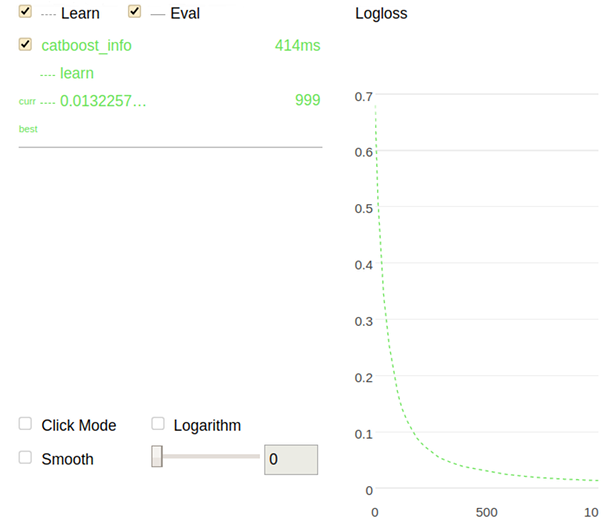## Read data from the specified directory only using MetricVisualizer

``````import catboost

w = catboost.MetricVisualizer('/path/to/trains/1')
w.start()``````

Refer to the MetricVisualizer section for details.

## Gather data from the specified directory only

1. Train a model from the root of the file system (/):
``````from catboost import CatBoostClassifier

cat_features = [0,1,2]

train_data = [["a", "b", 1, 4, 5, 6],
["a", "b", 4, 5, 6, 7],
["c", "d", 30, 40, 50, 60]]

train_labels = [1,1,0]

model = CatBoostClassifier(iterations=20,
loss_function = "CrossEntropy",
train_dir = "crossentropy")

model.fit(train_data, train_labels, cat_features)
predictions = model.predict(train_data)``````
2. Plot a chart using the information regarding the previous training (from the crossentropy directory):
``````import catboost

w = catboost.MetricVisualizer('/crossentropy/')
w.start()``````

The following is a chart plotted with Jupyter Notebook for the given example.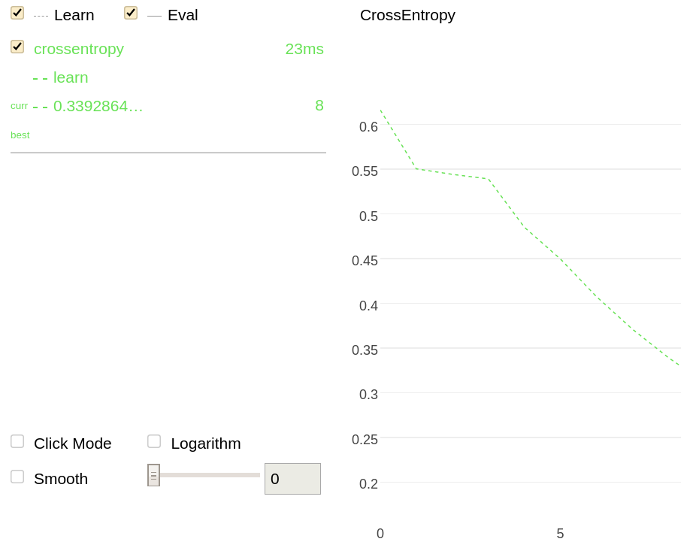## Gather and read data from all subdirectories of the specified directory using MetricVisualizer

``````import catboost

w = catboost.MetricVisualizer('/path/to/trains', subdirs=True)
w.start()``````

Any data located directly in the /path/to/trains folder is ignored. The information is read from its' subdirectories only.

Refer to the MetricVisualizer section for details.

## Gather and read data from all subdirectories

1. Train two models from the root of the file system (/):
1. ``````from catboost import CatBoostClassifier

cat_features = [0,1,2]

train_data = [["a", "b", 1, 4, 5, 6],
["a", "b", 4, 5, 6, 7],
["c", "d", 30, 40, 50, 60]]

train_labels = [1,1,0]

model = CatBoostClassifier(iterations=20,
loss_function = "CrossEntropy",
train_dir = "crossentropy")

model.fit(train_data, train_labels, cat_features)
predictions = model.predict(train_data)``````
2. ``````from catboost import CatBoostClassifier

cat_features = [0,1,2]

train_data = [["a", "b", 1, 4, 5, 6],
["a", "b", 4, 5, 6, 7],
["c", "d", 30, 40, 50, 60]]

train_labels = [1,1,0]

model = CatBoostClassifier(iterations=20,
train_dir = "logloss")

model.fit(train_data, train_labels, cat_features)
predictions = model.predict(train_data)``````
2. Plot charts using the information from all subdirectories (crossentropy and logloss) of the root of the file system:
``````import catboost

w = catboost.MetricVisualizer('/', subdirs=True)
w.start()``````

The following is a chart plotted with Jupyter Notebook for the given example.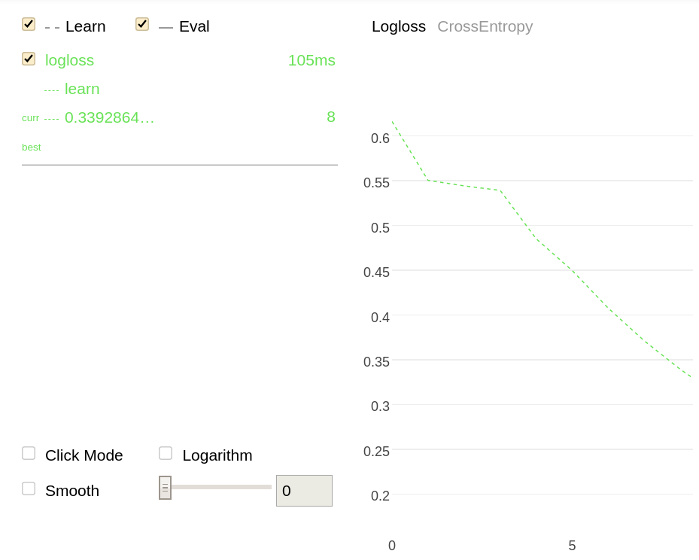## Perform cross-validation

Perform cross-validation on the given dataset:

``````from catboost import Pool, cv

cv_data = [["France", 1924, 44],
["USA", 1932, 37],
["Switzerland", 1928, 25],
["Norway", 1952, 30],
["Japan", 1972, 35],
["Mexico", 1968, 112]]

labels = [1, 1, 0, 0, 0, 1]

cat_features = 

cv_dataset = Pool(data=cv_data,
label=labels,
cat_features=cat_features)

params = {"iterations": 100,
"depth": 2,
"loss_function": "Logloss",
"verbose": False}

scores = cv(cv_dataset,
params,
fold_count=2,
plot="True")
``````

The following is a chart plotted with Jupyter Notebook for the given example.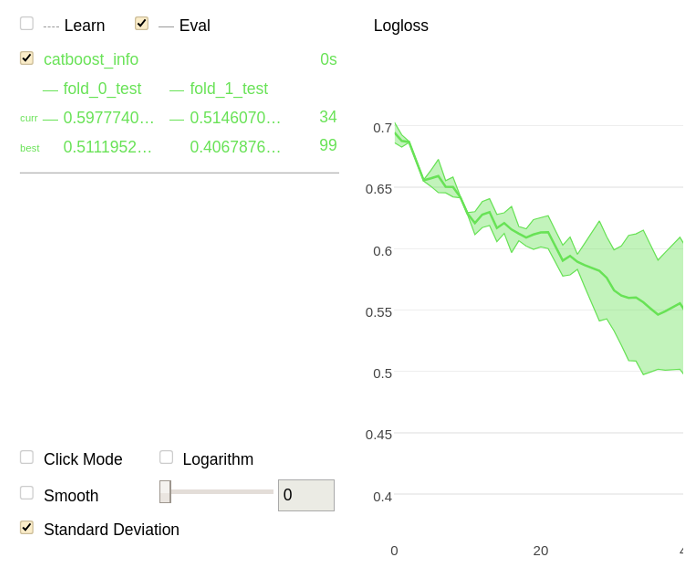Use the following code in Jupyter Notebook:

``````from catboost import CatBoost
import numpy as np

train_data = np.random.randint(1, 100, size=(100, 10))
train_labels = np.random.randint(2, size=(100))

model = CatBoost()

grid = {'learning_rate': [0.03, 0.1],
'depth': [4, 6, 10],
'l2_leaf_reg': [1, 3, 5, 7, 9]}

grid_search_result = model.grid_search(grid,
X=train_data,
y=train_labels,
plot=True)``````

The following is a chart plotted with Jupyter Notebook for the given example.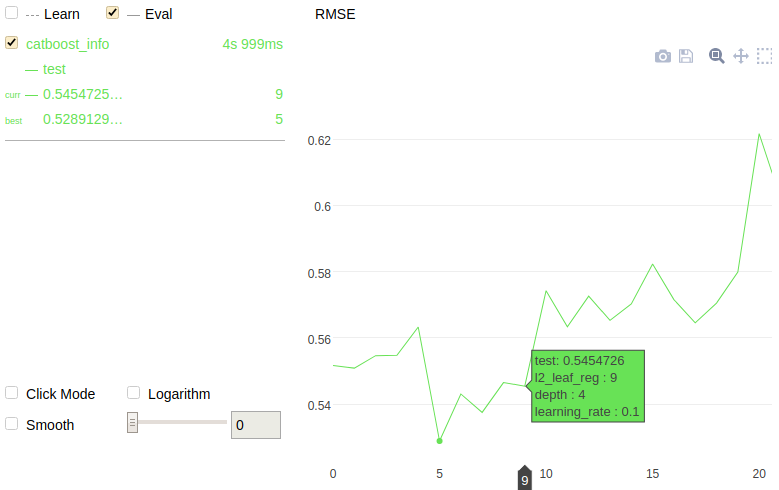``````from catboost import CatBoost

train_data = [[1, 4, 5, 6],
[4, 5, 6, 7],
[30, 40, 50, 60],
[20, 30, 70, 60],
[10, 80, 40, 30],
[10, 10, 20, 30]]
train_labels = [10, 20, 30, 15, 10, 25]
model = CatBoost()

grid = {'learning_rate': [0.03, 0.1],
'depth': [4, 6, 10],
'l2_leaf_reg': [1, 3, 5, 7, 9]}

randomized_search_result = model.randomized_search(grid,
X=train_data,
y=train_labels,
plot=True)``````

The following is a chart plotted with Jupyter Notebook for the given example.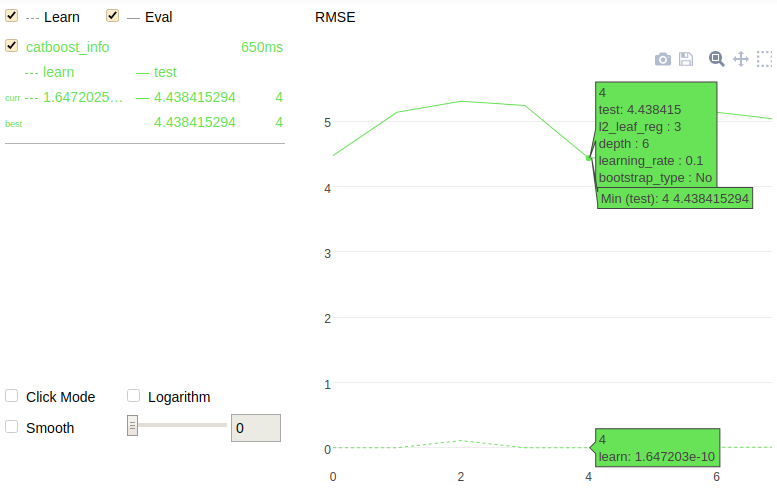## Feature statistics

А set of statistics for the chosen feature can be calculated and plotted.

Use one of the following methods to do this:

Calculate and plot a set of statistics for the chosen feature.

An example of plotted statistics: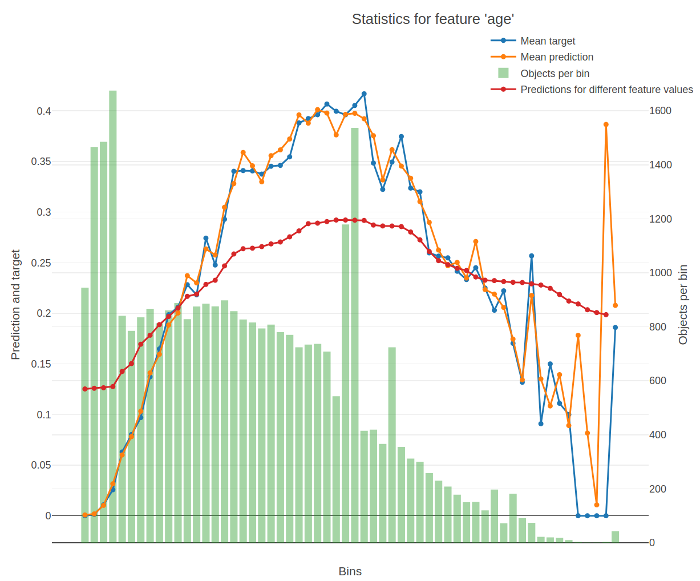The X-axis of the resulting chart contains values of the feature divided into buckets. For numerical features, the splits between buckets represent conditions (feature < value) from the trees of the model. For categorical features, each bucket stands for a category.

The Y-axis of the resulting chart contains the following graphs:

• Average target (label) value in the bucket.

• Average prediction in the bucket.

• Number of objects in the bucket.

• Average predictions on varying values of the feature.

To calculate it, the value of the feature is successively changed to fall into every bucket for every input object. The value for a bucket on the graph is calculated as the average for all objects when their feature values are changed to fall into this bucket.

The return value of the function contains the data from these graps.

The following information is used for calculation:

• Trained model
• Dataset
• Label values
Restriction.
• Only models with one-hot encoded categorical features are supported. Set the one_hot_max_size parameter to a large value to ensure that one-hot encoding is applied to all categorical features in the model.
• Multiclassification modes are not supported.